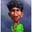Related Tags

javascript
programming
community creator

# Introduction to recursion in JavaScriptSubha Chanda

Recursion is an essential topic in the area of functional programming, but newbie programmers often find it challenging to understand what it is and how it works. So, I’ll try to make recursion easy for you or, at least, try to clarify your basic understanding of the topic. Let’s get started 😁.

## What is Recursion?

If you search “recursion” in Google you’ll get this message every time, “Did you mean: recursion”. And, when you click on the Did you mean link, it’ll show you the same thing again. But this is what recursion actually means, calling something again and again.In terms of computer programming, a recursive function is a function that calls itself until it satisfies some exit condition. Otherwise, we’ll be stuck into an infinite loop or, in case of JavaScript, the call stack will overflow. Let’s see an example to understand it better:

We get the factorial of a number if we continue multiplying the number with a value smaller than it until we reach 1. For example, the factorial for number 3 would be, 3 * 2 * 1 = 6.

Here’s a function that returns the factorial of a number:

function factorial(r) {
if(r <= 0) return 1;
else return r*factorial(r-1);
}
console.log(factorial(3)); //6

We are passing the number with the parameter r.

### How is this a recursive function?

If you look at the else condition, you’ll find that it is calling the function itself until it matches the exit condition, r <= 0. You might imagine that the same can be achieved using a loop, and you would be right.

Here is the same function using a loop:

function factorial(r) {
let factor = 1;
for(let i = r; i > 1; i--) {
factor *= i;
}
return factor;
}
console.log(factorial(3)); //6

Sometimes, in the case of such small functions, using a loop is the better choice. In JavaScript, it is generally cheaper to run a loop than it is to call a function multiple times, and the time complexity will be higher with a recursive function. Still, there are multiple instances where loops are not worth it. They can make the function more complex, and any recursive function can be implemented using loops. Now that we know what a recursive function is, let’s move on to building a recursive function by ourselves.

## Creating a recursive function

One of the most important things to keep in mind while working with a recursive function is to declare a termination condition. Otherwise, our function will overflow the call stack.

How do we declare a termination condition? Most simply, we can put it inside an if statement.

Example:

Suppose we want to write a recursive function that returns the sum of a given range. This means that if we pass 3, it will output the sum of the range from 1 to 3, i.e., 1 + 2 + 3 = 6.

Step 1: Declare a function name. It will take a single parameter that will specify the range. Feel free to use arrow functions if you want.

function sumRange(r){

}


Step 2: Declare a termination statement. When do we want this function to exit? When our range is 0, right?

function sumRange(r) {
if(r <= 0) return 0;
}


Step 3: Your logic is where the magic happens.

What do we want to achieve here? We want to do (current range + (range-1)) in a loop until our range becomes 0. We are getting the current range from r. How do we get the (range-1)? We call the function again and again by passing the parameter as (r-1).

function sumRange(r) {
if(r <= 0) return 0;
return r+sumRange(r-1);
}


## The function flow:

1. First, we call our function by passing the value 3.

sumRange(3);

2. The above call runs the function. It first checks the if statement and, if it finds it to be false, it’ll continue to work with the else. At else, we return 3 + the value of sumRange(3-1).

return 3 + sumRange(2);

3. The above logic also works here. Except that, this time, our range is 2. So, it’ll return 2 + sumRange(2-1).

return 2 + sumRange(1);

4. The same thing happens again. Now, the range is 1, so the returning sumRange will be (1-1).

return 1 + sumRange(0);


When the range becomes 0, our if the condition will run and return 0.

Now that all our function calls have returned a value, everything will start to unwind. The innermost function will return first.

So, sumRange(0) will return 0.

sumRange(1) will return 1 + sumRange(0) or, 1 + 0 = 1

sumRange(2) will return 2 + sumRange(1) or, 2 + 1 + 0 = 3

sumRange(3) will return 3 + sumRange(2) or, 3 + 2 + 1 + 0 = 6 and we get the answer 6 and we are done.

If you read the article to this point, a big thank you 😊. I hope you get some basic understanding of how recursion works and how to write a recursive function.

RELATED TAGS

javascript
programming
community creator

CONTRIBUTORSubha Chanda
RELATED COURSES

View all Courses

Keep Exploring

Learn in-demand tech skills in half the time